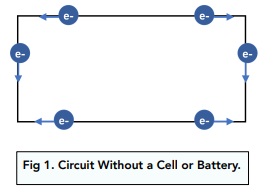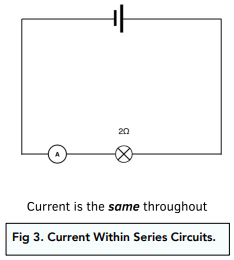# Electrical Charge

## Electrical Charge

• An electric current is a flow of charge. A circuit has many electrons (charges) flowing around. This is a current – a ‘flow of charge’.Electrical Charge

• A potential difference is needed for charge to flow. If you have an ordinary metal wire without a cell or battery, the electrons move randomly, and there is no net flow of charge. When a cell or battery – a potential difference / voltage – is applied to the metal wire, the electrons move away from the negative terminal and towards the positive terminal. There is a net flow of charge – an electric current.
• Current in metals is the flow of negatively charged electrons. Electrons can move from one atom to another, forming a sea of delocalised electrons.

• The current can be measured in amperes (A). The value for current,  in amperes (A), is the rate of flow of charge. It tells us how fast the charge is flowing through the circuit. The faster the flow of electrical charge, the larger the current. Similarly, the slower the flow of electrical charge, the smaller the current.
• A current is constant in a closed loop. A current has the same value at any point in a single closed loop of a circuit. Once we have learnt about series and parallel circuits this will make more sense. Within series circuits, the current is same throughout. Within parallel circuits, the current may vary between different branches (loops), but within each branch (loop) the current is constant.Electrical Charge